## Figures index

#### Lidong Wang, Cheryl Ann Alexander

American Journal of Systems and Software. 2014, 2(4), 94-100 doi:10.12691/ajss-2-4-3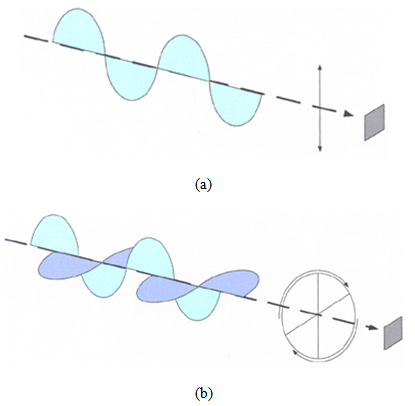• Figure 1. Classification of polarization: (a) linear polarization and (b) circular polarization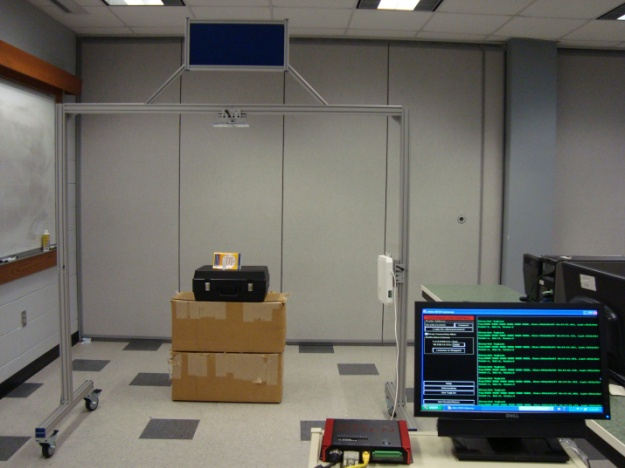• Figure 2. The BlueBean RFID Development Lab System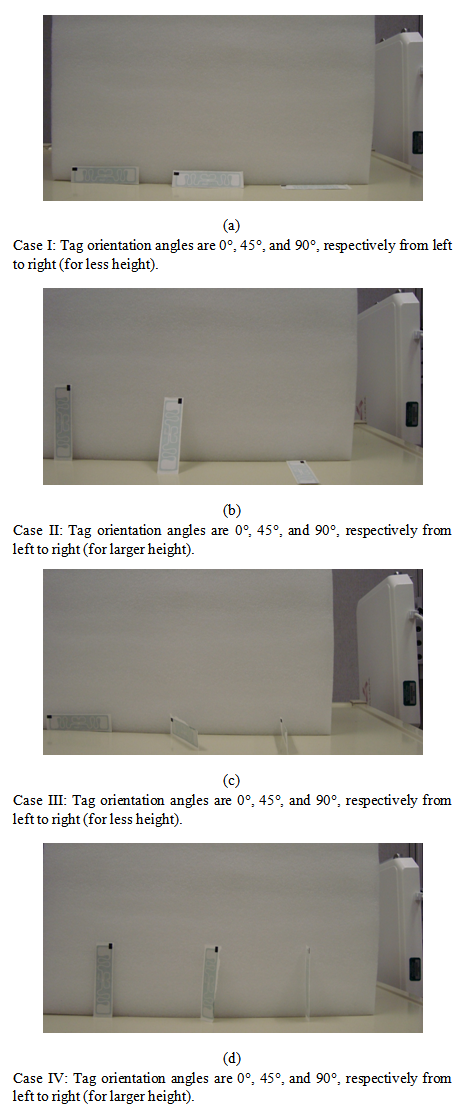• Figure 3. Tag orientations and angles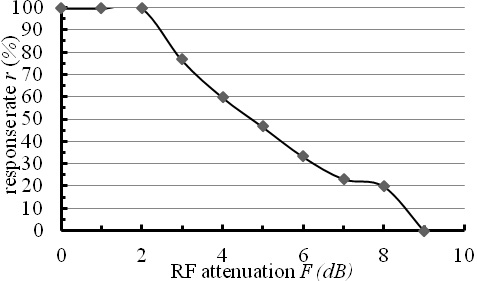• Figure 4. The response rate at various RF attenuation values (distance = 1200 mm)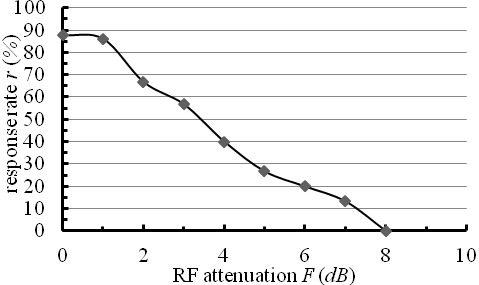• Figure 5. The response rate at various RF attenuation values (distance = 1260 mm)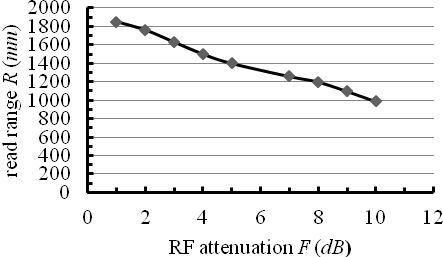• Figure 6. Obtained maximum RF attenuation F (given the read range R) using the first method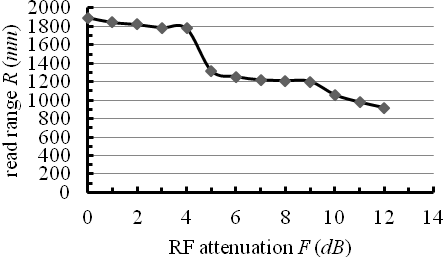• Figure 7. Obtained read range R (given the RF attenuation F) using the second method